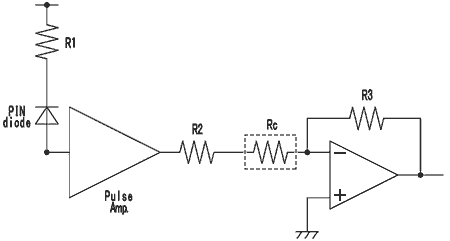# Products# Thermo-Sensitive Devices and Thermistors 1

## Thermo-sensitive devices, where to use

There are several types of such resistors that change resistance with the temperature. As an example, to switch control an equipment to a preset temperature, negative temperature coefficient (NTC) thermistor NT73, is used because it has a merit in sensitivity rather than linearity.

(Application example) Thermistor as a sensor to switch(on/off) control IC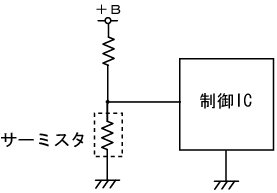Another example to use such a device is a temperature compensator. Linear positive temperature coefficient (PTC) resistor LP73 LT73 is conveniently used to linearly control the circuits, devices, etc. The resistor as a sensor and the device to be controlled should be thermally tightly coupled.

(Application example)LP73 LT73 is used as the bias resistor and output load resistor for temperature compensation of high frequency amplifiers. (We recommend LT73V for automotive applications.)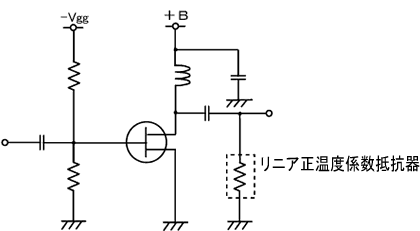## Temperature compensation of high frequency amplifiers

This is the example of mobile power stage amplifiers. When the temperature of the FET is raised, drain current will be increased. The positive temperature coefficient (PTC) resistor LP73 LT73(Rc in the figure) is coupled to the FET to increase the resistance value. Then the bias voltage is modified in such a way to decrease the drain current.
Thus the drain current is kept stable by the negative feedback.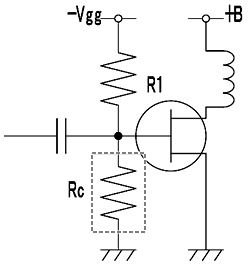## Temperature compensation of Hall elements

A hall element is a sensor, sensitive to the magnetic field. It is used as the non-contact current probe for oscilloscope, a non-contact potentiometer, etc.. For a GaAs type element, however, it has a temperature coefficient of around -600×10-6/K（max.）, which needs to be compensated.
A typical application circuit is shown. The Hall element is fed with a constant current and a differential voltage will be derived proportional to the applied magnetic field. This voltage is amplified and just compensated by the Rc, which is the specifiable linear positive temperature coefficient (PTC) resistor LT73 We recommend LT73V for automotive applications.

Temperature compensation of Hall Element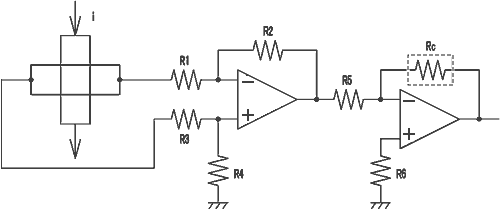## Temperature compensation of Optical pick-up

The sensitivity of a PIN photo-diode device is affected so greatly by the temperature that it is a prerequisite to be compensated. An example of a photo pick-up is shown in the figure, where the linear positive temperature coefficient resistor LP73 LT73 is used.
R1 is for reverse bias current to the PIN photo-diode, and Rc adjust the gain of the amplifier by the equation G=R3/(R2+Rc).
This equation means that the effect of compensation can be adjusted less sensitively in the direction to be weaken by the series resistor R2.

Note that Rc should be thermally close coupled to the PIN photo-diode. We recommend LT73V for automotive applications.

Temperature compensation of Optical pick-up